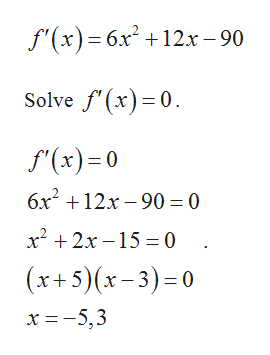# Consider the function f(x)=2x^3+6x^2-90x+8, [-5,4]find the absolute minimum value of this function.find the absolute maximum value of this function.

Question
6 views

Consider the function f(x)=2x^3+6x^2-90x+8, [-5,4]

find the absolute minimum value of this function.

find the absolute maximum value of this function.

check_circle

Step 1

The function is given by,

Step 2

Differentiatte with respe...help_outlineImage Transcriptionclosef'(x) 6x212x -90 Solve f'(x)0 f(x)0 6x212x-90= 0 x2 2x-15 0 (x+5)(x-3)0 x 5,3 fullscreen

### Want to see the full answer?

See Solution

#### Want to see this answer and more?

Solutions are written by subject experts who are available 24/7. Questions are typically answered within 1 hour.*

See Solution
*Response times may vary by subject and question.
Tagged in

### Other